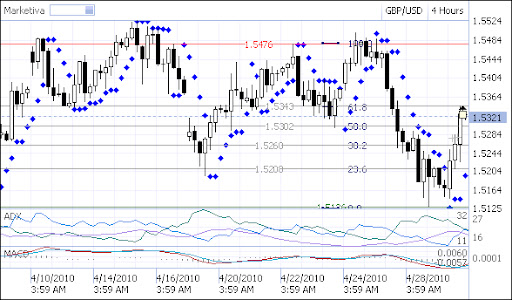Exchange Blog Cryptocurrency Blog# Using Fibonacci retracement for Predicting Limit Price

## Recommended Posts

Using Fibonacci retracement for Predicting Limit Price

Come let us play around with Fibonacci retracement

Indicators of this one is my favorite indicator of the Fibonacci itself I think is very interesting. Fibonacci sequence is a very beautiful series. The more we explore, the more the uniqueness of the sequence found in this seemingly simple. Arguably, the Fibonacci retracement is my first love of all indicators that I know ... hehehe

Ok, let's see ... how to use Fibonacci retracement wrote to analyze price movements.

In accordance with the basic philosophy of the Fibonacci itself, we try to observe the pattern of something that looks random, in this case the price movement of a pair. In something that looks random, it turns out we can find certain patterns that were "observed" in nature by everything in nature. Hmm ... if we discussed about how amazing his Fibonacci sequence, may be one book or one movie alone ... hehehe ...

Yeah never mind ... I will not discuss in detail about the Fibonacci sequence and all the mystery that is in it. If you are interested to know this series, please search in search engines ...

We immediately discussed how the use of this series on the chart ... ok?

The first step to use the Fibonacci retracement is, we must indentified swing or wave that occurred. That is why, Fibonacci often associated with other wave theories such as Elliot Wave. While we postpone a discussion of the Elliot Wave. We focus first on how interesting Fibonacci Retracemet in our chart

Ok, next ...

Once we know the Swing High-Low, we trick Fibonacci retracement. Adjust the level 0 and 100 on the Fibonacci with high-low that has been identified.

For more details, let us examine the following chart:In the chart above, I installed indikatir Parabolic SAR, MACD and ADX and draw the Fibonacci retracement of the swing were identified. High and Low at 1.5476 at 1.5126. Fibonacci retracement I pull with 0 at low and 100 in high.

Now let us consider how to comply with the price movement patterns formed by the levels is Fibonacci retracement. Look at how the price of trying to penetrate each of those levels. Notice how the price of business through price level 1.5208 and 1.5260 and 1.5343. Price touch many times those limits in order to try to penetrate it. After the pierced, the price will be advanced to the next level.

By understanding these behaviors, we can use prices in these Fibonacci levels as our profit targets, such as examples of positions that I took where I use the benchmark level of 61.8 Fibo at 1.5343 as a target price of profit. The position I take it only as an example. TP coincidence that I took only about 20 pips hell .. hehehe ...

Basically, we can use the Fibonacci retracement at all time frames. But, it is advisable to use for the hourly time frame upwards. It's nothing ... , if we use 5-minute time frame, and each time the wave is formed then we draw Fibo again, then when we start trading? .... Hehehe ...

Anyway, we can also use Fibonacci retracement as a benchmark to determine support and resistant replacement pivot point

##### Share on other sites

• 1 year later...

How this it works...i dont understand

##### Share on other sites

• 11 months later...

market move formed like a wave and there will be always higher low or lower low in every trend movement. these movement is retracement or pullback. and to figuring out in which level these higher low and lower low will move, traders can use fibonacci indicator. this indicator able to predicting the price level accurately and if we observe, the price will always react in every of fibonacci level.

## Join the conversation

You can post now and register later. If you have an account, sign in now to post with your account.×   Pasted as rich text.   Paste as plain text instead

Only 75 emoji are allowed.